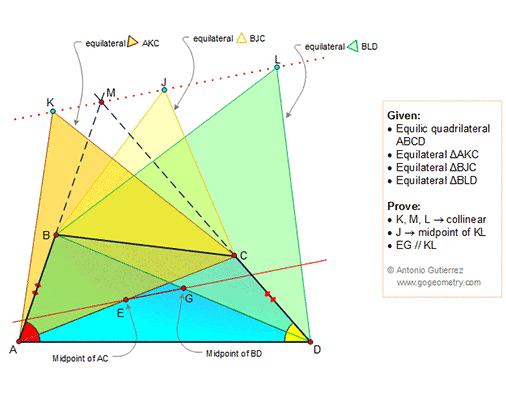Saturday, July 28, 2018

Geometry Problem 1371: Equilic Quadrilateral, 120 Degrees, Congruence, Midpoint, Diagonal, Equilateral Triangle, Parallel, Collinearity

Geometry Problem. Post your solution in the comment box below.
Level: Mathematics Education, High School, Honors Geometry, College.

Details: Click on the figure below.1 comment:

1.https://photos.app.goo.gl/uab3srEzcKiFoa6n7

1. Per the results of previous problems we have AJD and ENG are equilateral triangles
Quadrilateral AKMC is cyclic => ∠ (KMA)= ∠ (KCA)= 60
Quadrilateral AMJD is cyclic => ∠ (JMD)= ∠ (JAD)= 60
∠ (KMJ)= 60+60+60= 180 => K,M, J are collinear
Quadrilateral BMLD is cyclic => ∠ (LMD)= ∠ (LBD)= 60
Since ∠ (LBD)= ∠ (JMD)= 60 => M, J, L are collinear and K, M, J, L are collinear
2. Triangle DLJ congruent to DBA ( case SAS) => JL= AB
Triangle AKJ congruent to ACD ( case SAS) => KJ=CD
Since AB= CD => J is the midpoint of KL
3. Since NE //PM and NG // MD => triangle PMQ similar to ENG ( case AA) => PMQ is equilateral
And ∠ (JMQ)= ∠ (MQP)= 60 so EG//KL